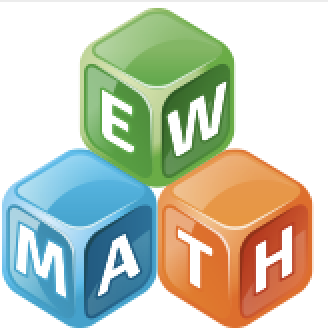## Available courses

### Singapore Math Essentials in K-1 (Jul 12 - Aug 9, 2020)

Participants will learn strategies for teaching essential concepts necessary to build strong number sense foundation in K-1. Educators will learn how to make effective use of instructional materials. Math in Focus, Primary Mathematics, and Dimensions Math’s teacher editions, textbooks, workbooks and other relevant materials will be used. Acquired knowledge will be directly applicable to understanding and implementing all Singapore-based math programs. Topics covered include understanding cardinality of numbers, decomposition and composition of numbers, number bonds, activities that develop number sense and facts fluency, place value, and teaching addition & subtraction with meaning. This course is aligned with Next Generations Standards.### Intro to Singapore Math Section K-1 (Aug 2 - Aug 29)

Introduction to Singapore Math, K-6

This course will provide participants with the foundation for understanding and implementing Singapore based math programs such as Primary Mathematics, Math in Focus, and Eureka Math in Kindergarten through sixth grades. This course will introduce participants to the theoretical framework and constructivist pedagogy of the Singapore based mathematics programs. The course provides an overview of establishing Singapore Math PLC’s,  CPA-based instruction, drawing of bar models, and lesson planning using a Singapore text.### Intro to Singapore Math Section 2-5 (Aug 2 - Aug 29)

Introduction to Singapore Math, K-6

This course will provide participants with the foundation for understanding and implementing Singapore based math programs such as Primary Mathematics, Math in Focus, and Eureka Math in Kindergarten through sixth grades. This course will introduce participants to the theoretical framework and constructivist pedagogy of the Singapore based mathematics programs. The course provides an overview of establishing Singapore Math PLC’s,  CPA-based instruction, drawing of bar models, and lesson planning using a Singapore text.